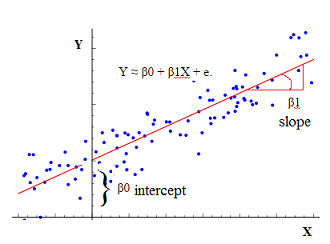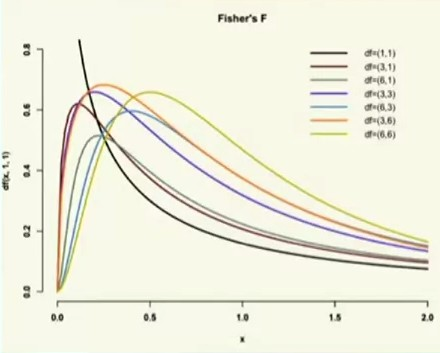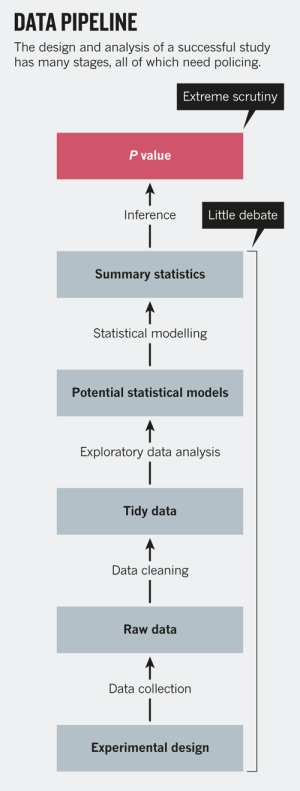# Statistics - t-distributions

The t-distributions are used to calculate the p-value (basically you look it up in a table, nowadays software will compute it).

It's basically a normal random variable except for small numbers of samples.

They are a Family of t distribution depending on sample size. They are all sampling distributions.

As your sample size get smaller, your t distribution gets a little wider which means you need a larger t value to get out into the extremes to get a low p-value because the p value is the probability of obtaining these data or more extreme (The extremes) outcomes given the assumption that the null hypothesis is true.

In contrast to the z distribution, there are multiple t distributions. The correct distribution to use is determined by the Degrees of freedom (df).

The null hypothesis being true will gives a t-value of zero.

The p-value comes from the t-value and its corresponding t-distribution which depends on sample size. The p-value is just the area under the t distribution curve at that particular t value and beyond.

So if you:

• are way out in the extremes, you're going to have a low p-value. You'll reject the NULL.
• fall around the middle, then you'll have a high p-value. You'll retain the NULL.

p-value < 0.5 means less than 5% of the distribution are in the extreme positive tail of the t-distribution or in the extreme negative tail of the t-distribution.

Discover MoreStatistics - (Univariate|Simple|Basic) Linear Regression

A Simple Linear regression is a linear regression with only one predictor variable (X). Correlation demonstrates the relationship between two variables whereas a simple regression provides an equation...Statistics - (dependent|paired sample) t-test

A dependent t-test is appropriate when: we have the same people measured twice. the same subject are been compared (ex: Pre/Post Design) or two samples are matched at the level of individual subjects...Statistics - Confidence Interval

The definition of a confidence interval says that under repeated experiments 95% of the time this confidence interval will contain the true statistic (mean, ...). if we started the whole experiment over...Statistics - F-distributions

Like the t-test and family of t-distributions, the F-test has a family of F-distributions The family of F-distributions depends on: the Number of subjects per group the Number of groups ANOVA...Statistics - P-value

Fisher found with the a P-value a way of judging the “significance” of experimental data entirely objectively. Fisher choose the talismanic figure 0.05 because it was mathematically convenient and...Statistics - independent t-test

A dependent t-test is appropriate when you want to compare two independent samples. (two completely different groups of entities). For examples: if we want to compare one condition to another as men...